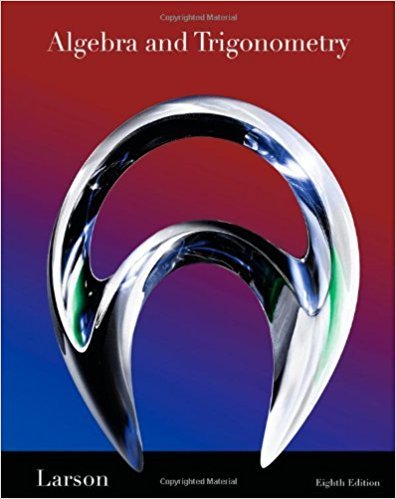×
×

# Solutions for Chapter 9: Systems of Equations and Inequlities## Full solutions for Algebra and Trigonometry | 8th Edition

ISBN: 9781439048474Solutions for Chapter 9: Systems of Equations and Inequlities

Solutions for Chapter 9
4 5 0 416 Reviews
21
3
##### ISBN: 9781439048474

This expansive textbook survival guide covers the following chapters and their solutions. This textbook survival guide was created for the textbook: Algebra and Trigonometry, edition: 8. Algebra and Trigonometry was written by and is associated to the ISBN: 9781439048474. Since 116 problems in chapter 9: Systems of Equations and Inequlities have been answered, more than 48888 students have viewed full step-by-step solutions from this chapter. Chapter 9: Systems of Equations and Inequlities includes 116 full step-by-step solutions.

Key Math Terms and definitions covered in this textbook
• Back substitution.

Upper triangular systems are solved in reverse order Xn to Xl.

• Basis for V.

Independent vectors VI, ... , v d whose linear combinations give each vector in V as v = CIVI + ... + CdVd. V has many bases, each basis gives unique c's. A vector space has many bases!

• Cholesky factorization

A = CTC = (L.J]))(L.J]))T for positive definite A.

A sequence of steps (end of Chapter 9) to solve positive definite Ax = b by minimizing !x T Ax - x Tb over growing Krylov subspaces.

• Echelon matrix U.

The first nonzero entry (the pivot) in each row comes in a later column than the pivot in the previous row. All zero rows come last.

• Exponential eAt = I + At + (At)2 12! + ...

has derivative AeAt; eAt u(O) solves u' = Au.

• Factorization

A = L U. If elimination takes A to U without row exchanges, then the lower triangular L with multipliers eij (and eii = 1) brings U back to A.

• Free variable Xi.

Column i has no pivot in elimination. We can give the n - r free variables any values, then Ax = b determines the r pivot variables (if solvable!).

• Fundamental Theorem.

The nullspace N (A) and row space C (AT) are orthogonal complements in Rn(perpendicular from Ax = 0 with dimensions rand n - r). Applied to AT, the column space C(A) is the orthogonal complement of N(AT) in Rm.

• Inverse matrix A-I.

Square matrix with A-I A = I and AA-l = I. No inverse if det A = 0 and rank(A) < n and Ax = 0 for a nonzero vector x. The inverses of AB and AT are B-1 A-I and (A-I)T. Cofactor formula (A-l)ij = Cji! detA.

• Kirchhoff's Laws.

Current Law: net current (in minus out) is zero at each node. Voltage Law: Potential differences (voltage drops) add to zero around any closed loop.

• Length II x II.

Square root of x T x (Pythagoras in n dimensions).

• Linear combination cv + d w or L C jV j.

• Normal equation AT Ax = ATb.

Gives the least squares solution to Ax = b if A has full rank n (independent columns). The equation says that (columns of A)·(b - Ax) = o.

• Positive definite matrix A.

Symmetric matrix with positive eigenvalues and positive pivots. Definition: x T Ax > 0 unless x = O. Then A = LDLT with diag(D» O.

• Projection p = a(aTblaTa) onto the line through a.

P = aaT laTa has rank l.

• Semidefinite matrix A.

(Positive) semidefinite: all x T Ax > 0, all A > 0; A = any RT R.

• Similar matrices A and B.

Every B = M-I AM has the same eigenvalues as A.

• Simplex method for linear programming.

The minimum cost vector x * is found by moving from comer to lower cost comer along the edges of the feasible set (where the constraints Ax = b and x > 0 are satisfied). Minimum cost at a comer!